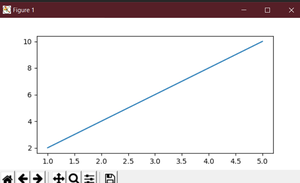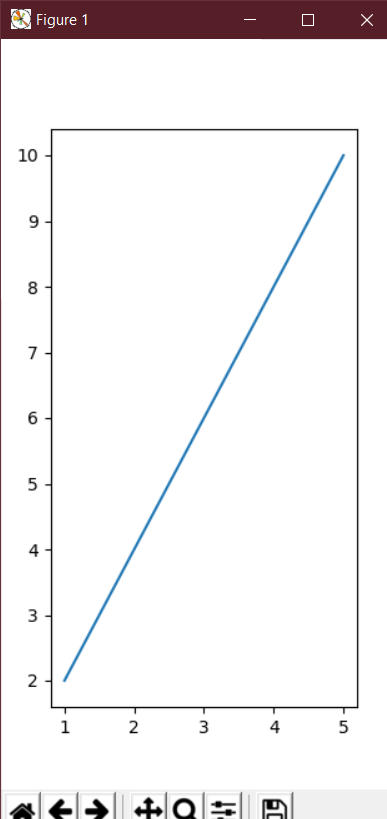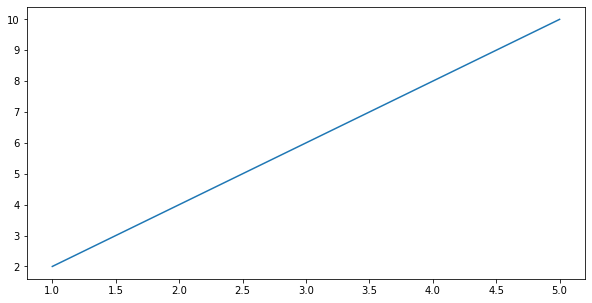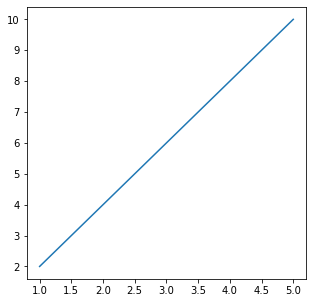Open in App
Not now

# How to change the size of figures drawn with matplotlib?

• Difficulty Level : Medium
• Last Updated : 17 Nov, 2022

In this article, we will see how to change the size of the Figures In Matplotlib in Python.

## How to use it for plotting?

The main purpose of Matplotlib is to create a figure representing data. The use of visualizing data is to tell stories by curating data into a form easier to understand, highlighting the trends and outliers. We can populate the figure with all different types of data, including axes, a graph plot, a geometric shape, etc. “When” we plot graphs we may want to set the size of a figure to a certain size. You may want to make the figure wider in size, taller in height, etc.

## Increase or decrease the plot size in Matplotlib

This can be achieved by an attribute of Matplotlib known as figsize. The figsize attribute allows us to specify the width and height of a figure in-unit inches.

### Syntax of matplotlib.pyplot

Syntax:

import matplotlib.pyplot as plt

figure_name = plt.figure(figsize=(width, height))

The figsize attribute is a parameter of the function figure(). It is an optional attribute, by default the figure has the dimensions as (6.4, 4.8). This is a standard plot where the attribute is not mentioned in the function.

Normally each unit inch is of 80 x 80 pixels. The number of pixels per unit inch can be changed by the parameter dpi, which can also be specified in the same function.

Approach:

• We create a variable plt_1, and set it equal to, plt.figure(figsize=(6,3)).
• This creates a figure object, which has a width of 6 inches and 3 inches in height.
• The values of the figsize attribute are a tuple of 2 values.

## Python3

 `# We start by importing matplotlib``import` `matplotlib.pyplot as plt` `# Plotting a figure of width 6 and height 3``plt_1 ``=` `plt.figure(figsize``=``(``6``, ``3``))` `# Let's plot the equation y=2*x``x ``=` `[``1``, ``2``, ``3``, ``4``, ``5``]` `# y = [2,4,6,8,10]``y ``=` `[x``*``2` `for` `x ``in` `x]` `# plt.plot() specifies the arguments for x-axis``# and y-axis to be plotted``plt.plot(x, y)` `# To show this figure object, we use the line,``# fig.show()``plt.show()`

Output:This works if you’re using a python IDE other than Jupiter notebooks. If you are using  Jupiter notebooks, then you would not use, plt.show(). Instead, you would specify in the Code right after importing matplotlib, %matplotlib inline.

### Example 2: Change Figure Size in Matplotlib

To see the dynamic nature of figure sizing in Matplotlib, now we have to create a figure with the dimensions inverted. The height will now be double the size of the width.

## Python3

 `# We start by importing matplotlib``import` `matplotlib.pyplot as plt` `# Plotting a figure of width 3 and height 6``plt_1 ``=` `plt.figure(figsize``=``(``3``, ``6``))` `# Let's plot the equation y=2*x``x ``=` `[``1``, ``2``, ``3``, ``4``, ``5``]` `# y = [2,4,6,8,10]``y ``=` `[x``*``2` `for` `x ``in` `x]` `# plt.plot() specifies the arguments for``# x-axis and y-axis to be plotted``plt.plot(x, y)` `# To show this figure object, we use the line,``# fig.show()``plt.show()`

Output:### Example 3: Set the Height and Width of a Figure in Matplotlib

In this example, we will see that instead of simply using figsize we can also set the height and width of the plot using set_figheight() and set_figwidth() functions.

## Python3

 `# We start by importing matplotlib``import` `matplotlib.pyplot as plt` `# Plotting a figure of width 10 and height 5``fig ``=` `plt.figure()` `fig.set_figheight(``5``)``fig.set_figwidth(``10``)` `# Let's plot the equation y=2*x``x ``=` `[``1``, ``2``, ``3``, ``4``, ``5``]` `# y = [2,4,6,8,10]``y ``=` `[x``*``2` `for` `x ``in` `x]` `# plt.plot() specifies the arguments for x-axis``# and y-axis to be plotted``plt.plot(x, y)` `# To show this figure object, we use the line,``# fig.show()``plt.show()`

Output:### Example 4:Set the Height and Width of a Figure in Inches.

Here, we will see another example of setting figure size in inches using set_size_inches(5, 5).

## Python3

 `# We start by importing matplotlib``import` `matplotlib.pyplot as plt` `# Plotting a figure of width 5 and height 5``fig ``=` `plt.figure()` `fig.set_size_inches(``5``, ``5``)` `# Let's plot the equation y=2*x``x ``=` `[``1``, ``2``, ``3``, ``4``, ``5``]` `# y = [2,4,6,8,10]``y ``=` `[x``*``2` `for` `x ``in` `x]` `# plt.plot() specifies the arguments for x-axis``# and y-axis to be plotted``plt.plot(x, y)` `# To show this figure object, we use the line,``# fig.show()``plt.show()`

Output:My Personal Notes arrow_drop_up